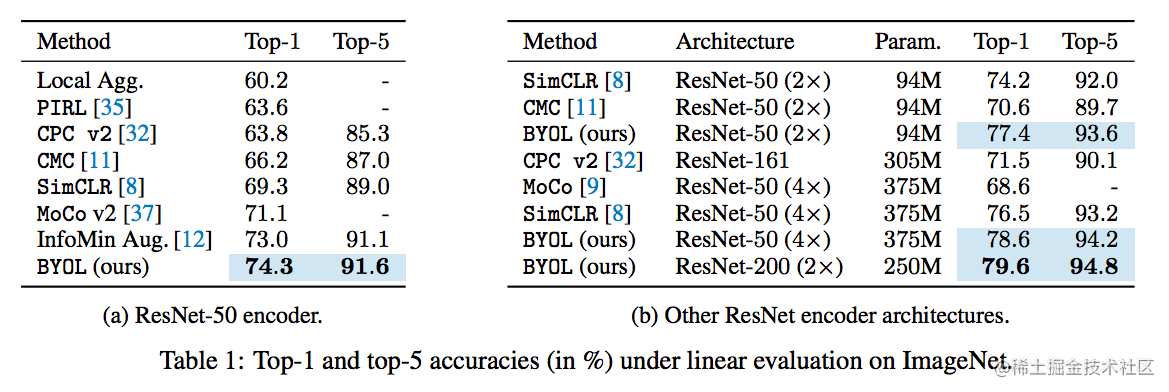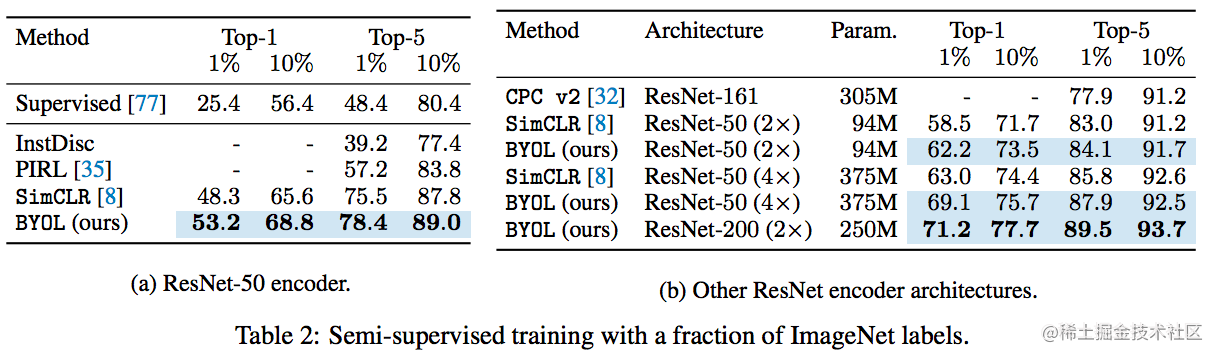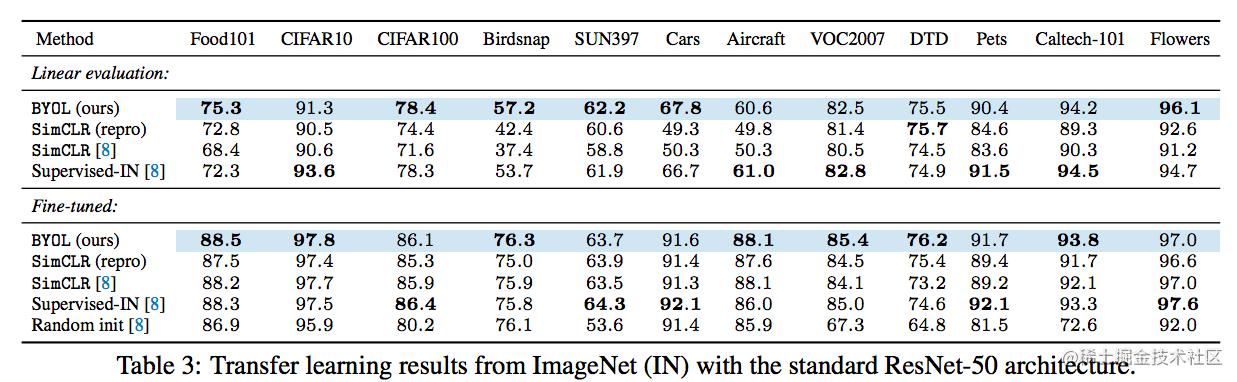﻿ 自监督SOTA框架 | BYOL(优雅而简洁) | 2020_其他_开心洋葱网
• 欢迎访问开心洋葱网站，在线教程，推荐使用最新版火狐浏览器和Chrome浏览器访问本网站，欢迎加入开心洋葱 QQ群
• 为方便开心洋葱网用户，开心洋葱官网已经开启复制功能！
• 欢迎访问开心洋葱网站，手机也能访问哦~欢迎加入开心洋葱多维思维学习平台 QQ群
• 如果您觉得本站非常有看点，那么赶紧使用Ctrl+D 收藏开心洋葱吧~~~~~~~~~~~~~！
• 由于近期流量激增，小站的ECS没能经的起亲们的访问，本站依然没有盈利，如果各位看如果觉着文字不错，还请看官给小站打个赏~~~~~~~~~~~~~！

# 自监督SOTA框架 | BYOL(优雅而简洁) | 2020

2416次浏览
• 文章原创自微信公众号「机器学习炼丹术」
• 作者：炼丹兄
• 联系方式：微信cyx645016617

• 论文名称：“Bootstrap Your Own Latent: A New Approach to Self-Supervised Learning”
• 论文链接：https://arxiv.org/pdf/2006.07733.pdf

## 1 数学符号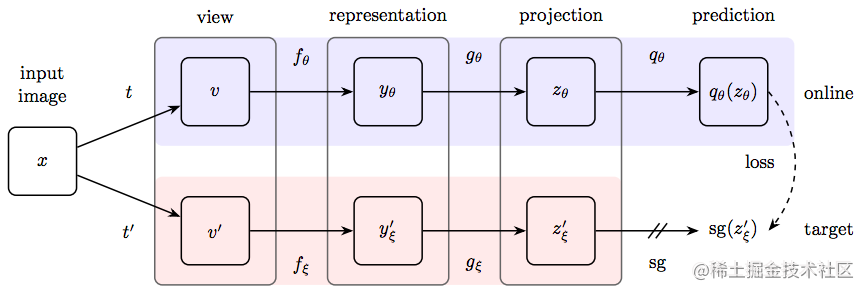• online network：用$$\theta$$来表示online network的参数，包含，encoder$$f_{\theta}$$,projector$$g_{\theta}$$和predictor$$q_\theta$$
• target netowrk:使用$$\xi$$来表示参数，也有$$f_{\xi}$$$$g_{\xi}$$,但是没有predictor。

$$\xi\leftarrow \tau\xi + (1-\tau)\theta$$

## 2 损失函数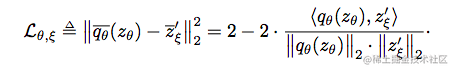$$L^{BYOL}_{\theta,\xi}=L_{\theta,\xi} + \widetilde{L}_{\theta,\xi}$$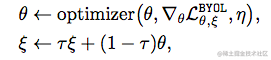## 3 细节部分

### 3.1 图像增强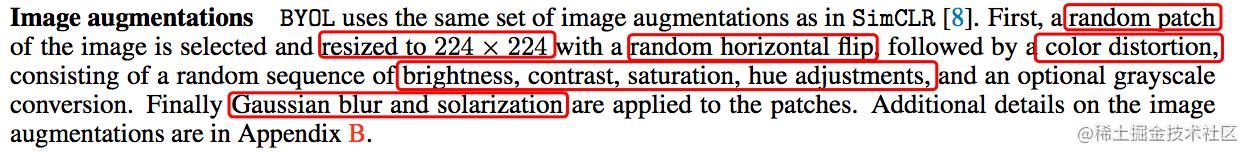### 3.2 结构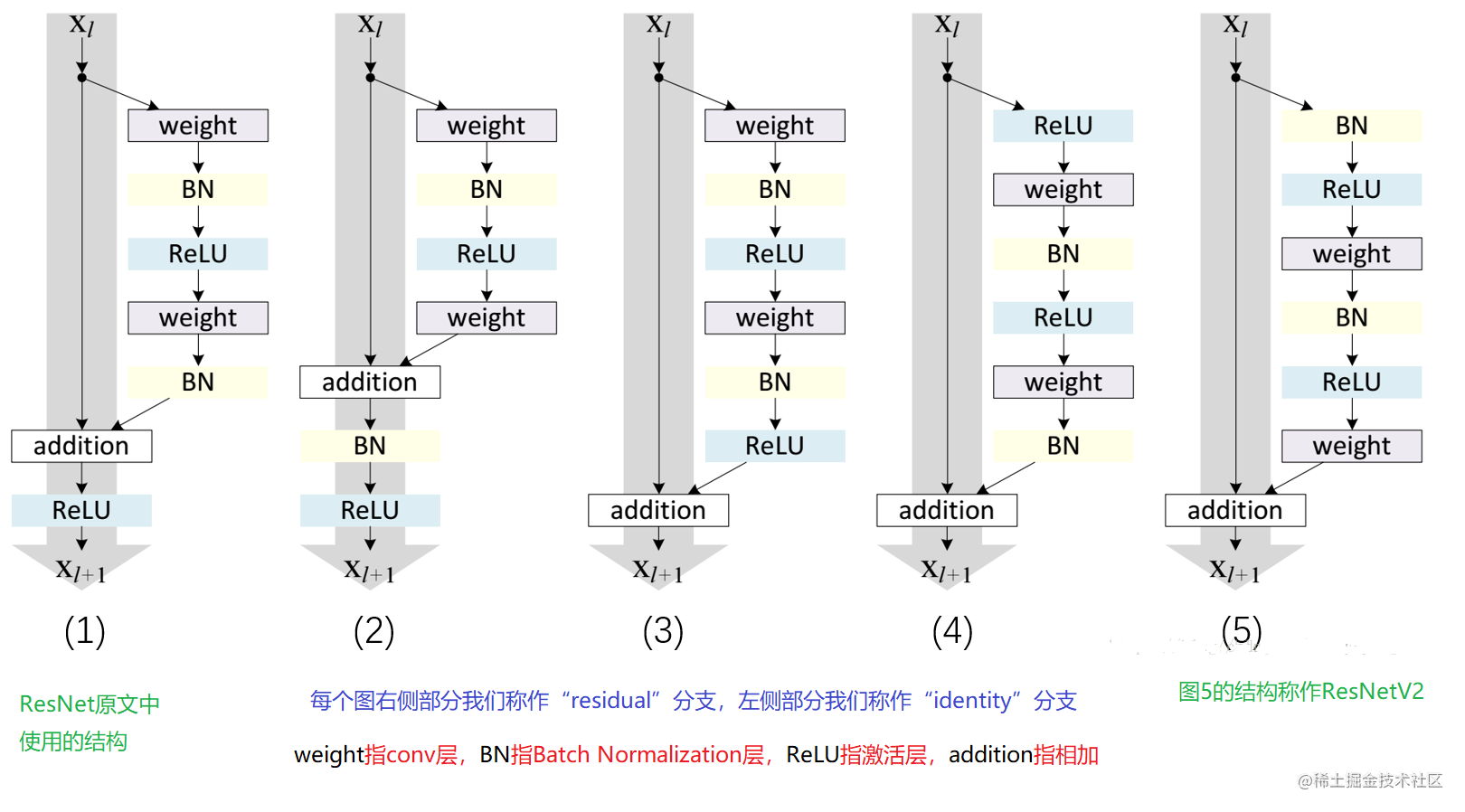### 3.3 优化器

$$\tau=1-(1-\tau_{base})(\cos\frac{\pi k}{K}+1)\frac{1}{2}$$

k is current training step and K is maximum training steps.

### 3.4 财力

batchsize为4096，分布在512个TPU v3的核，训练encoder大约需要8个hour。

## 4 模型评估